Courses

# Test: Sequences And Series (Competition Level) - 3

## 25 Questions MCQ Test Mathematics (Maths) Class 11 | Test: Sequences And Series (Competition Level) - 3

Description
This mock test of Test: Sequences And Series (Competition Level) - 3 for JEE helps you for every JEE entrance exam. This contains 25 Multiple Choice Questions for JEE Test: Sequences And Series (Competition Level) - 3 (mcq) to study with solutions a complete question bank. The solved questions answers in this Test: Sequences And Series (Competition Level) - 3 quiz give you a good mix of easy questions and tough questions. JEE students definitely take this Test: Sequences And Series (Competition Level) - 3 exercise for a better result in the exam. You can find other Test: Sequences And Series (Competition Level) - 3 extra questions, long questions & short questions for JEE on EduRev as well by searching above.
QUESTION: 1

### The sum of integers from 1 to 100 that are divisible by 2 or 5 is

Solution:

Set of numbers divisible by 2 are 2,4,6,...,100
Set of numbers divisible by 5 are 5,10,15,...,100
Set of numbers divisible by 10 are 10,20,30,...,100
Now, sum of numbers divisible by 2 are given by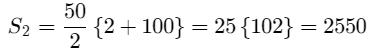Sum of numbers divisible by 5 are given by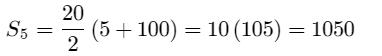Sum of numbers divisible by 10 are given by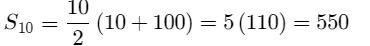Hence required sum = S2 ​ +S5 ​ −S10 = 2550 + 1050 − 550 = 3050

QUESTION: 2

### Consider an A.P. with first term `a' and the common difference `d'. Let Sk denote the sum of its first K terms. If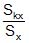is independent of x, then

Solution:

Given, first term =a, common difference =d
By using Sn = n/2 [2a+(n−1)d] we have,
∴ Skx / Sx = ​ kx/2​(2a+(kx−1)d) / x/2(2a+(x+1)d)
⇒Skx / Sx ​= k(2a+(kx−1)d) / (2a+(x+1)d)
⇒Skx / Sx = k(2a−d+kxd) / 2a+xd−d
Now this will be independent of x only when 2a=d or a = d/2

QUESTION: 3

### The next term of the sequence, 2, 6, 12, 20, …..is

Solution:

nth term of given sequence is n(n+1)
so the 5th term is 5(5+1)=30

QUESTION: 4

If A, G & H are respectively te A.M., G.M. & H.M. of three positive numbers a, b, & c then the equation whose roots are a, b & c is given by

Solution: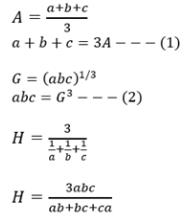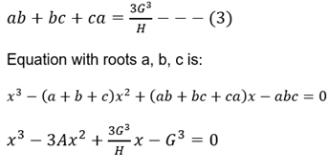QUESTION: 5

If ax = by = cz = dt and a, b, c, d are in G.P., then x, y, z, t are in

Solution:
QUESTION: 6

If xi > 0, i = 1, 2,....,50 ans x1 + x2 + ....+x50 = 50, then the minimum value of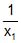++ ......+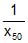equal to

Solution:
QUESTION: 7

If a, a1, a2, a3, ....... , a2n, b are in A.P. and a, g1, g2, g3,.....g2n, b are in G.P. and h is the harmonic mean of a and b, then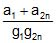+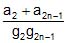+........ .....+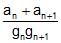, is equal to

Solution:
QUESTION: 8

One side of an equilateral triangle is 24 cm. The mid-points of its sides are joined to form another triangle whose mid-points are in turn joined to form still another triangle. This process continues indefinitely. Then the sum of the perimeters of all the trianlges is

Solution:
QUESTION: 9

If a1, a2....an are in A.P. with common difference d > 0, then the sum of the series (sin d) [cosec a1 cosec a2 + cosec a2 cosec a3 +..... ......+ cosec an – 1 cosec an]

Solution:

A₁ , A₂ , A₃ , .......An are in AP and common difference is d
so, d = A₂ - A₁ = A₃ - A₂ = A₄ - A₃ =...... =An - An-1 -------(1)

Now, sind[cosecA₁.cosecA₂ + cosecA₂.cosecA₃ + cosecA₃.cosecA₄ ...........+ cosecAn-1.cosecAn ]
⇒ sind[1/sinA₁.sinA₂ + 1/sinA₂.sinA₃ + 1/sinA₃.sinA₄ + .........+ 1/sinAn₋₁.sinAn]
⇒ sin d/sinA₁.sinA₂ + sind/sinA₂.sinA₃ + sind/sinA₃.sinA₄ + .......+sind/sinAn₋₁.sinAn
⇒ sin(A₂ - A₁)/sinA₁.sinA₂ + sin(A₃ - A₂)/sinA₂.sinA₃ + sin(A₄ - A₃)/sinA₃.sinA₄ + .......+ sin(An-1 - An)/sinAn-1.sinAn [ from equation (1)
⇒ (sinA₁.cosA₂ - cosA₁.sinA₂)/sinA₁.sinA₂ + (sinA₂.cosA₃ - cosA₂.sinA₃)/sinA₂.sinA₃ +.........+ (sinAn-1.cosAn - cosAn-1.sinAn)/sinAn-1.sinAn
⇒cotA₂ - cotA₁ + cotA₃ - cotA₂ + cotA₄ - cotA₃ + .........cotAn - cotAn-1
⇒cotAn - cotA₁

QUESTION: 10

An infinite GP has first term x and sum 5, then x belongs to

Solution:
QUESTION: 11

If S1, S2, S3 are the sums of first n natural numbers, their squares, their cubes respectively, then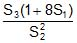is equal to

Solution:
QUESTION: 12

If a1, a2, ...., an are in HP, then the expression a1a2 + a2a3 + ....+ an – 1 an is equal to

Solution:
QUESTION: 13

If x2 + 9y2 + 25z2 = xyz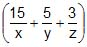, then x, y and z are in

Solution:
QUESTION: 14

The sum of all possible products of first n natural numbers taken two by two is

Solution:
QUESTION: 15

If G1 and G2 and two geometric means and A is the arithmetic means inserted between two positive numbers then the value of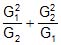is

Solution:
QUESTION: 16

{an} and {bn} are two sequences given byan =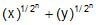and bn =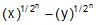for all n ∈ N. The value of a1 a2 a3...... an is equal to

Solution:
QUESTION: 17

If 12 + 22 + 32 + ..... + 20032 = (2003) (4007) (334) and (1) (2003) + (2) (2002) + (3) (2001) +.... ....+ (2003) (1) = (2003) (334) (x)., then x equals

Solution:

for 2003 terms the summation is = (2003)(2004)(4007) / 6 = (2003)(4007)(334)
Now,
(1)(2003)+(2)(2002)+(3)(2001)+....(2003)(1) =
= [ 2004(2003)(2003 + 1) / 2 ] - [ (2003)(4007)(334) ]
= [ (1002)(2003)(2004) ] - [ (2003)(4007)(334) ]
= 3 [(334)(2003)(2004) ] - [ (2003)(4007)(334)]
= (2003)(334)( 3*2004 - 4007)
= (2003)(334)(2005)

QUESTION: 18

Sum of first fifteen terms of series 5+10+20+… is

Solution:

a= 5 r=2
arn-1 = 5(2)n-1
Sn = a(rn -1)/r-1
= 5(215-1)/2-1
= 5(32768-1)1
= 98301

QUESTION: 19

The common difference d of the A.P. in which T7 = 9 and T1T2T7 is least is

Solution:
QUESTION: 20

The H.M. between two numbers is , their A.M. is A and G.M. is G. If 2A + G2 = 26, then the numbers are

Solution:
QUESTION: 21

If 1, 2, 3.... are first terms; 1, 3, 5..... are common differences and S1, S2, S3.... are sums of n terms of given p AP's; then S1 + S2 + S3 + .... + Sp is equal to

Solution:
*Multiple options can be correct
QUESTION: 22

For the A.P. given by a1, a2,...............an,..........., the equations satisfied are

Solution:
*Multiple options can be correct
QUESTION: 23

If sum of the infinite G.P., p,1,1/p2 ,1/p3,...... is 9 /2, the value of p is

Solution:
*Multiple options can be correct
QUESTION: 24

If positive numbers a, b, c are in A.P. and a2, b2, c2 are in H.P., then

Solution:
*Multiple options can be correct
QUESTION: 25

If the arithmetic mean of two positive numbers a & b (a > b) is twice their geometric mean, then a : b is

Solution: